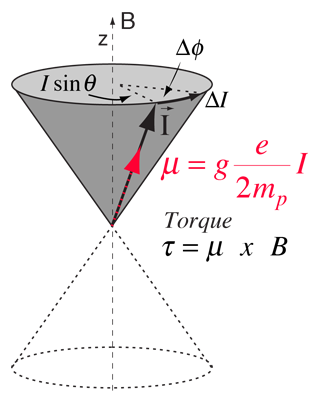# Larmor Frequency

When a magnetic moment is placed in a magnetic field it will tend to align with the field. Classically, a magnetic moment can be visualized as a current loop and the influence toward alignment can be described as the torque on the current loop exerted by the magnetic field. The idea of the magnetic moment as a current loop can be extended to describe the magnetic moments of orbital electrons, electron spins and nuclear spins. In each case the magnetic moment is associated with the angular momentum, and a torque can be identified which tends to align the magnetic moment with the magnetic field. In the nuclear case, the angular momentum involved is the intrinsic angular momentum I associated with the nuclear spin.

When you have a magnetic moment directed at some finite angle with respect to the magnetic field direction, the field will exert a torque on the magnetic moment. This causes it to precess about the magnetic field direction. This is analogous to the precession of a spinning top around the gravity field. The torque can be expressed as the rate of change of the nuclear spin angular momentum I and equated to the expression for the magnetic torque on the magnetic momentwhich when put in derivative form gives a precession angular velocityIt can also be visualized quantum mechanically in terms of the quantum energy of transition between the two possible spin states for spin 1/2. This can be expressed as a photon energy according to the Planck relationship. The magnetic potential energy difference is hυ = 2μB. The angular frequency associated with a "spin flip", a resonant absorption or emission involving the spin quantum states is often written in the general form ω = gB where g is called the gyromagetic ratio (sometimes the magnetogyric ratio). Note that this frequency is a factor of two higher than the one above because of the spin flip with energy change ΔE = 2μB. This nuclear spin transition for nuclei placed in a magnetic field is the basis for nuclear magnetic resonance (NMR)
 Larmor precession of electron orbital magnetic moment
Index

Nuclear Spectra Concepts

 HyperPhysics***** Nuclear R Nave
Go Back